## A research team conducted a study showing that approximately 10% of all businessmen who wear ties wear them so tightly that they actually re

Question

A research team conducted a study showing that approximately 10% of all businessmen who wear ties wear them so tightly that they actually reduce blood flow to the brain, diminishing cerebral functions. At a board meeting of 15 businessmen, all of whom wear ties, what are the following probabilities?
a. at least one tie is too tight 0.794
b. more than two ties are too tight 0.913
c. no tie is too tight 0.087
d. at least 13 ties are not too tight 0.816

in progress 0
6 months 2021-07-31T00:59:02+00:00 1 Answers 74 views 0

a) 0.794 = 79.4% probability that at least one tie is too tight.

b) 0.184 = 18.4% probability that more than two ties are too tight.

c) 0.206 = 20.6% probability that no tie is too tight.

d) 0.816 = 81.6% probability that at least 13 ties are not too tight.

Step-by-step explanation:

For each tie, there are only two possible outcomes. Either they are too tight, or they are not too tight. The probability of a businessmen wearing a tie too tight is independent of any other businessmen, which means that the binomial probability distribution is used to solve this question.

Binomial probability distribution

The binomial probability is the probability of exactly x successes on n repeated trials, and X can only have two outcomes.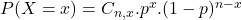In which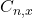is the number of different combinations of x objects from a set of n elements, given by the following formula.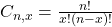And p is the probability of X happening.

10% of all businessmen who wear ties wear them so tightly

This means that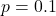This means that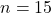a. at least one tie is too tight

This is: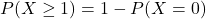In which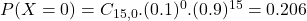Then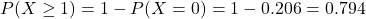0.794 = 79.4% probability that at least one tie is too tight.

b. more than two ties are too tight 0.913

This is: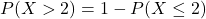In which: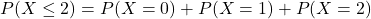. So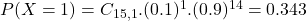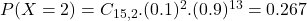Then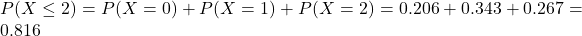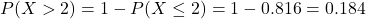0.184 = 18.4% probability that more than two ties are too tight.

c. no tie is too tight

This is P(X = 0). So0.206 = 20.6% probability that no tie is too tight.

d. at least 13 ties are not too tight 0.816

At most 2 are too tight, which is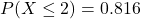, found in option b.

0.816 = 81.6% probability that at least 13 ties are not too tight.